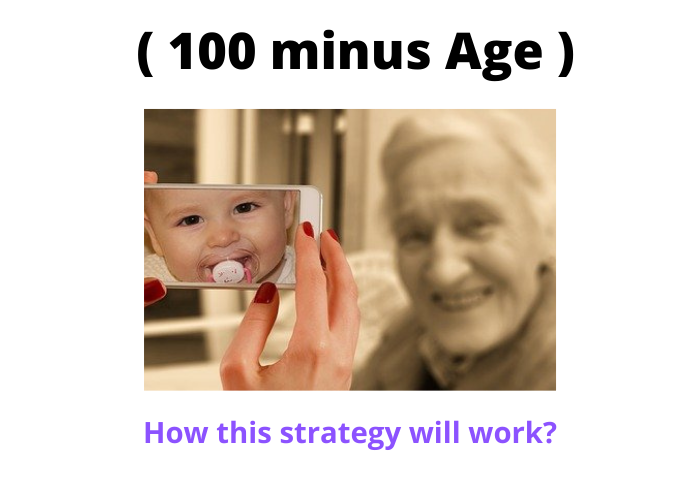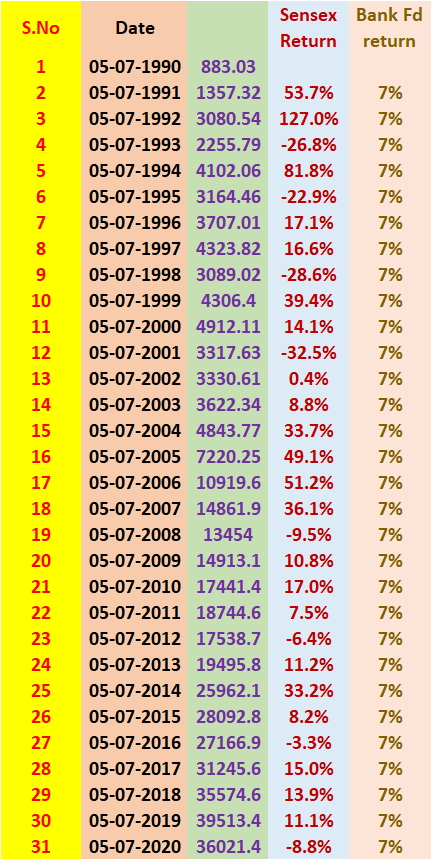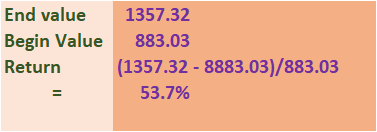# (100 minus Age) – How this strategy will protect your wealth?Yes, (100 minus age ) strategy will protect your wealth If you are investing stock market.

If you are investing in the stock market, you may have heard or read that you should take more risks when you are young.

In addition,  You may have you should take less risk when you are getting older.

Here,  High Risk means investing more money into the stock market and Low risk means less money into the stock market.

## What is ( 100 minus age ) Investment Strategy?…

A layman can not understand when to invest and when to exit the stock market.

But if there is any thumb rule, then the layman also can implement the stock market investment easily.

( 100 minus age ) is such kind of thumb rule,

In this rule, You should invest in equity ( stock market) using a formula.

For example, your age is 30 years and you want to invest Rs.100 in the stock market.

But As per the ( 100 – age ) thumb rule, you should not invest Rs.100 entirely in equity ( stock market ).

In addition, You should deduct your age ( 30 ) from the total amount that you are looking to invest.

i.e 100 – 30 = 70 ( amount to be invested in equity ).

So, you can invest Rs.70 ( 70% of Rs.100) only in the stock market at age 30 if your age is 30 years.

Similarly, if you age 31, then the amount that can be invested in equity is as shown below.

i.e 100 minus 31 = 69 ( 69%) will be invested in the stock market.

In this way, you have to decrease the equity exposure every year with an increase in age.

One more important thing is to invest the money left after investing in the stock market will be invested in the r asset class (low-risk asset class ) like bank FD.

i.e Rs. 31 ( 31%) in the above example.

Moreover, You need to increase your bank fd exposure with an increase in your age.

### Case Study for ( 100 minus age ) investment strategy…

Let’s assume that Mr. X would like to invest Rs.1,00,000 in the stock market.

In addition, his present age is 30, and he would like to continue his investment till he retires at age 60.

Now, using the above data we can back-test the equity investment with ( 100 minus age ) strategy to protect Mr. X’s wealth.

And at the same to create good wealth for Mr.X.

For this purpose, I took the Sensex historical values for the purpose of back-testing for the equity investing part.

The Sensex values for the past 30 years in the month of July is as shown in the below image.

In addition, the image contains Sensex’s historical yearly returns and also bank FD returns.You can see in the above image The Sensex’s historical prices on 5th July of every year from 1990 to 2020.

By using the below formulae, I have calculated the Sensex’s yearly returns.

Absolute Return = ( End Value – Begin Value ) / Begin Value.

Understand absolute return calculation using the below image.You can see in the above image that the Sensex has delivered a return of 53.7% from July 1990 to July 1991.

Similarly, I have calculated the returns for the rest of the years.

We all know that the present Bank Fd interest rates are around 5.6%.

But the last 30 years Bank Fd interest as an average is around is 7%. ( from 1990 to 2020).

#### Now Let’s analyze what happened to your investment using the ( 100 minus age ) strategy…

You can see in the above image Mr. X’s age is 30 and he has invested Rs.70,000 ( 100 – age i.e 70% ) in the Sensex and the balance money in bank Fd in the year 1990.

As the Sensex has given 53.7% return from the year 1990 to 1991, Rs.70,000 investment had become Rs.1,07,598.

While at the same, The Bank Fd return is 7%, and Rs.30,000 had become Rs.32,100.

If you both the values, the total value is Rs.1,39,698.

Again, Mr.X has to invest the above money ( Rs.1,39,698) based on the same ( 100 – age ) strategy.

I.e 69% in the equity and 31% in Bank Fd.

So, 69% of Rs.1,39,698 = Rs.96,392.

And 31% of Rs.1,39,698 = Rs.43,306.

Here also you should calculate the total value after the end of the 2nd year for both Sensex investment and Bank Fd investment and you should add them to total investment value.

This process should continue till Mr. retires. ( i.e up to 60 years of age ).

You can see at the age of 60, Mr. X’s total investment had become 27.5 lakh.

##### What is Mr. X’s investment total value at age 60 if invested entre 1 lakh in Sensex only.?…

The Sensex value in July 1990 is 883.03 and in July 2020 is 36,021.4.

Here the Number of years between 1990 to 2020 is 30 years.

Hence, You can calculate the rate of return (CAGR) using formulae Rate = ( Fv/Pv)^(1/n)-1

Here, Fv = 36,021.4 and Pv = 883.03 and N = 30.

After putting the values in the formulae the CAGR = 13.16%.

Now, you can calculate the total investment value if invested 100% in the Sensex only.

and the formulae to be used is Future value ( FV) = Present Value*( 1+ Rate)^N.

Pv = Rs,1,00,000. R = 13.16% and N = 30.

After putting the values in the formulae, the future value is Rs.40,79,297.4 ( approximately 40 lakh ).

But it is very clear that the future value in ( 100 minus age ) strategy is only 27 lakh while in 100% equity investment strategy is 40 lakh.

are you think 100% equity investment is good?

No, it is not, I will tell you why?

###### Why ( 100 – age ) investment protects your wealth and it is good than a 100% equity investment strategy?…

If you are an equity investor or thinking to invest in equity, you should know that equity markets sometimes correct ( fall ) in a big way.

And these markets will take some time to recover.

In the year period 2008 to 2009, the Sensex had corrected ( fell ) nearly 69%.

So, if the equity market falls at age 60 for Mr.X, and in the same year, Mr. X needs the entire money.( inf invested 100% in equity).

Then, the investment value available for Mr. X after the fall is Rs.40,00,000*(1-69%) = Rs.12,00,000 ( approximately).

When it comes 100 – age investment strategy, Mr. X’s investment has two components.

One is equity and the second is Bank Fd.

At age 60, Sensex Investment for Mr. X  is 11 lakh ( approx) and Bank Fd is 17 Lakh ( approx ).

Every one of us knows, when the share market falls only our investment which we have invested in the Sensex will fall.

In 100 – age strategy, Mr. X has 11 lakh in equity ( Sensex).

So, if the Market or the Sensex falls by 69%, then only 11 lakh investment will fall not 17 investment in Bank Fd.

So, Rs.11,00,000 * ( 1- 69%  = 3.4 Lakh ( approx).

Hence, the total investment value of Mr. X after a 69% fall in 100 – age strategy is shown below.

Total Value is = 17 Lakh ( Bank Fd) + 3.4 ( Sensex investment after 69% fall) = 20.4 lakh.

So, it is very clear you have a higher investment value ( 20.4 lakh ) in 100 – age strategy than 12 lakh in 100% in the Sensex strategy when there is a big fall.

Please do not argue with me that Mr.X has lost 13 lakh for not investing 100% in the Sensex.

No one when share markets will fall, and when you are investing in equity markets you should manage the risks in it also.

###### What is the Conclusion about ( 100 minus age ) investment strategy?…

I compare this strategy with driving a bike wearing a helmet and 100% Sensex strategy with driving without a helmet.

If you met with an accident without wearing a helmet, the chances of survival are minimal.

While at the same with the helmet, the chances will increase significantly.

However, the Bank Fd rate that I have assumed is 7% average, But I take actual Fd rates every year, the end value may be much higher than that I have calculated.

In addition, this is the thumb rule for investing for the layman, there may be much better options or strategies available to invest if you have enough knowledge or if you take professional help like from Sebi Registered Investment Adviser.

So, it’s up to you to opt which strategy is best for you for creating wealth and at the same which protects wealth too.

Read How to beat 1 crore with 1 lakh investment in the article.

and also read about real estate vs equity investment which is best.

Are you giving Tithe – Then you will lose 55 lakh? How?

Share With Friends and Family